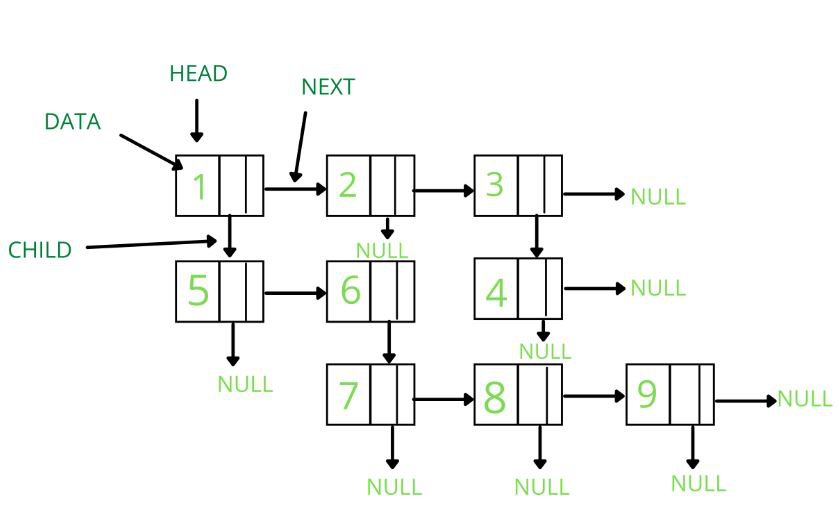• Difficulty Level : Medium
• Last Updated : 18 Aug, 2021

Multilevel Linked List is a 2D data structure that comprises several linked lists and each node in a multilevel linked list has a next and child pointer. All the elements are linked using pointers.Representation:
A multilevel linked list is represented by a pointer to the first node of the linked lists. Similar to the linked list, the first node is called the head. If the multilevel linked list is empty, then the value of head is NULL. Each node in a list consists of at least three parts:
1. Data.
2. Pointer to the next node.
3. Pointer to the child node.

Attention reader! Don’t stop learning now. Get hold of all the important DSA concepts with the DSA Self Paced Course at a student-friendly price and become industry ready.  To complete your preparation from learning a language to DS Algo and many more,  please refer Complete Interview Preparation Course.

In case you wish to attend live classes with experts, please refer DSA Live Classes for Working Professionals and Competitive Programming Live for Students.

Each node of a multilevel linked list is represented as:

class Node
{
public:
int data;
Node *next;
Node *child;
};

Below is the implementation of the multilevel linked list

## C++

 `// C++ program to implement``// a multilevel linked list` `#include ``using` `namespace` `std;` `// Representation of node``class` `Node {``public``:``    ``int` `data;``    ``Node* next;``    ``Node* child;``};` `// A function to create a linked list``// with n(size) nodes returns head pointer``Node* createList(``int``* arr, ``int` `n)``{``    ``Node* head = NULL;``    ``Node* tmp;` `    ``// Traversing the passed array``    ``for` `(``int` `i = 0; i < n; i++) {``        ``// Creating a node if the list``        ``// is empty``        ``if` `(head == NULL) {``            ``tmp = head = ``new` `Node();``        ``}``        ``else` `{``            ``tmp->next = ``new` `Node();``            ``tmp = tmp->next;``        ``}``        ``// Created a node with data and``        ``// setting child and next pointer``        ``// as NULL.``        ``tmp->data = arr[i];``        ``tmp->next = tmp->child = NULL;``    ``}``    ``return` `head;``}` `// To print the linked list``void` `printMultiLevelList(Node* head)``{``    ``// While head is not null``    ``while` `(head) {``        ``if` `(head->child) {``            ``printMultiLevelList(head->child);``        ``}``        ``cout << head->data << ``" "``;``        ``head = head->next;``    ``}``}` `// Driver code``int` `main()``{``    ``// Initializing the data in arrays(row wise)``    ``int` `arr1 = { 1, 2, 3 };``    ``int` `arr2 = { 5, 6 };``    ``int` `arr3 = { 4 };``    ``int` `arr4 = { 7, 8, 9 };` `    ``// creating Four linked lists``    ``// Passing array and size of array``    ``// as parameters``    ``Node* head1 = createList(arr1, 3);``    ``Node* head2 = createList(arr2, 2);``    ``Node* head3 = createList(arr3, 1);``    ``Node* head4 = createList(arr4, 3);` `    ``// Initializing children and next pointers``    ``// as shown in given diagram``    ``head1->child = head2;``    ``head1->next->next->child = head3;``    ``head2->next->child = head4;` `    ``// Creating a null pointer.``    ``Node* head = NULL;``    ``head = head1;` `    ``// Function Call to print``    ``printMultiLevelList(head);` `    ``return` `0;``}`

## Java

 `/*package whatever //do not write package name here */``import` `java.io.*;` `class` `GFG {``   ``public` `static` `class` `Node {``      ``int` `data;``        ``Node next;``        ``Node child;``    ``};` `    ``// A function to create a linked list``    ``// with n(size) nodes returns head pointer``    ``public` `static` `Node createList(``int` `arr[], ``int` `n)``    ``{``        ``Node head = ``null``;``        ``Node tmp = ``null``;` `        ``// Traversing the passed array``        ``for` `(``int` `i = ``0``; i < n; i++)``        ``{``          ` `            ``// Creating a node if the list``            ``// is empty``            ``if` `(head == ``null``) {``                ``tmp = head = ``new` `Node();``            ``}``            ``else` `{``                ``tmp.next = ``new` `Node();``                ``tmp = tmp.next;``            ``}``          ` `            ``// Created a node with data and``            ``// setting child and next pointer``            ``// as NULL.``            ``tmp.data = arr[i];``            ``tmp.next = tmp.child = ``null``;``        ``}``        ``return` `head;``    ``}` `    ``// To print the linked list``    ``public` `static` `void` `printMultiLevelList(Node head)``    ``{``      ` `        ``// While head is not null``        ``while` `(head != ``null``) {``            ``if` `(head.child != ``null``) {``                ``printMultiLevelList(head.child);``            ``}``            ``System.out.print(head.data + ``" "``);``            ``head = head.next;``        ``}``    ``}` `    ``// Driver code``    ``public` `static` `void` `main(String[] args)``    ``{``        ``int` `arr1[] = { ``1``, ``2``, ``3` `};``        ``int` `arr2[] = { ``5``, ``6` `};``        ``int` `arr3[] = { ``4` `};``        ``int` `arr4[] = { ``7``, ``8``, ``9` `};` `        ``// creating Four linked lists``        ``// Passing array and size of array``        ``// as parameters``        ``Node head1 = createList(arr1, ``3``);``        ``Node head2 = createList(arr2, ``2``);``        ``Node head3 = createList(arr3, ``1``);``        ``Node head4 = createList(arr4, ``3``);` `        ``// Initializing children and next pointers``        ``// as shown in given diagram``        ``head1.child = head2;``        ``head1.next.next.child = head3;``        ``head2.next.child = head4;` `        ``// Creating a null pointer.``        ``Node head = ``null``;``        ``head = head1;` `        ``// Function Call to print``        ``printMultiLevelList(head);``    ``}``}` `// This code is contributed by maddler.`

## C#

 `/*package whatever //do not write package name here */``using` `System;` `public` `class` `GFG {``   ``public` `class` `Node {``          ``public` `int` `data;``        ``public` `Node next;``        ``public` `Node child;``    ``};` `    ``// A function to create a linked list``    ``// with n(size) nodes returns head pointer``    ``public` `static` `Node createList(``int` `[]arr, ``int` `n)``    ``{``        ``Node head = ``null``;``        ``Node tmp = ``null``;` `        ``// Traversing the passed array``        ``for` `(``int` `i = 0; i < n; i++)``        ``{``          ` `            ``// Creating a node if the list``            ``// is empty``            ``if` `(head == ``null``) {``                ``tmp = head = ``new` `Node();``            ``}``            ``else` `{``                ``tmp.next = ``new` `Node();``                ``tmp = tmp.next;``            ``}``          ` `            ``// Created a node with data and``            ``// setting child and next pointer``            ``// as NULL.``            ``tmp.data = arr[i];``            ``tmp.next = tmp.child = ``null``;``        ``}``        ``return` `head;``    ``}` `    ``// To print the linked list``    ``public` `static` `void` `printMultiLevelList(Node head)``    ``{``      ` `        ``// While head is not null``        ``while` `(head != ``null``) {``            ``if` `(head.child != ``null``) {``                ``printMultiLevelList(head.child);``            ``}``            ``Console.Write(head.data + ``" "``);``            ``head = head.next;``        ``}``    ``}` `    ``// Driver code``    ``public` `static` `void` `Main(String[] args)``    ``{``        ``int` `[]arr1 = { 1, 2, 3 };``        ``int` `[]arr2 = { 5, 6 };``        ``int` `[]arr3 = { 4 };``        ``int` `[]arr4 = { 7, 8, 9 };` `        ``// creating Four linked lists``        ``// Passing array and size of array``        ``// as parameters``        ``Node head1 = createList(arr1, 3);``        ``Node head2 = createList(arr2, 2);``        ``Node head3 = createList(arr3, 1);``        ``Node head4 = createList(arr4, 3);` `        ``// Initializing children and next pointers``        ``// as shown in given diagram``        ``head1.child = head2;``        ``head1.next.next.child = head3;``        ``head2.next.child = head4;` `        ``// Creating a null pointer.``        ``Node head = ``null``;``        ``head = head1;` `        ``// Function Call to print``        ``printMultiLevelList(head);``    ``}``}` `// This code is contributed by shikhasingrajput`
Output
`5 7 8 9 6 1 2 4 3 `

My Personal Notes arrow_drop_up